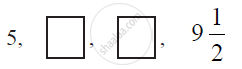# In the following APs, find the missing terms in the boxes - Mathematics

In the following APs, find the missing terms in the boxes :#### Solution

For this A.P.,

a = 5 and

a4 = 19/2

We know that, an = a + (n − 1) d

a4 = a + (4 - 1) d

19/2 = 5 + 3d

19/2-5 = 3d

9/2=3d

d = 3/2

a2 = a + (2 - 1) d

a2 = 5 + 3/2

a2 = 13/2

a3 = a + (3 - 1) d

a3 = 5 + 2×3/2

a3 = 8

Therefore, the missing terms are 13/2 and 8 respectively.

Concept: nth Term of an AP
Is there an error in this question or solution?
Chapter 5: Arithmetic Progressions - Exercise 5.2 [Page 106]

#### APPEARS IN

NCERT Class 10 Maths
Chapter 5 Arithmetic Progressions
Exercise 5.2 | Q 3.3 | Page 106
Share